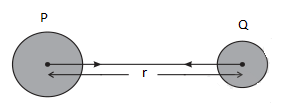# Gravitation

Notes Assignments

## Universal Law of Gravitation

• Universal Law of gravitation was formulated by Sit Issac Newton.
• It states that
Every body in universe attracts every other body with a force which is directly proportional to the product of their masses and inversely proportional to the square of distance between them. The force acts along the line joining the two bodies
• To understand that let us consider two objects of masses $M$ and $m$ that lie at a distance $r$ from each other as shown below in the figure• Let $F$ be the force of attraction between two objects $P$ and $Q$.
• Now according to the universal law of gravitation as stated earlier , this force $F$ is proportional to the product of masses of objects $P$ and $Q$ that is
$F \propto M \times m$              (1)
and the force is inversely proportional to the square of distance between these two objects that is
$F \propto \frac{1}{{{r^2}}}$             (2)
• Combining both equations (1) and (2) we get
$F \propto \frac{{Mm}}{{{r^2}}}$             (3)
or
$F = G\frac{{Mm}}{{{r^2}}}$             (4)
Here G is the constant known as Universal Gravitational constant.
• SI unit of gravitational constant G is $N{m^2}K{g^{ - 2}}$
• Value of G was first found by Henry Cavendish (1731-1810) and it is
$G = 6.673 \times {10^{ - 11}}N{m^2}K{g^{ - 2}}$

## Important Characteristics of Gravitational forces

• the gravitational force is a central force that is It acts along the line joining the centers of two bodies.
• it is a long range force. The gravitational force is effective even at large distances.
• It is a conservative force. This means that the work done by the gravitational force in displacing a body from one point to another is only dependent on the initial and final positions of the body and is independent of the path followed.
• Unlike electrostatic and magnetic forces, the gravitational force is always attractive.

## Why is this Universal Law of Gravitation so important

• It is the force that is responsible for binding us to Earth.
• It is responsible for the motion of moon around the earth
• It is responsible for the motion of planets around the sun
• The tides formed by rising and falling of water level in the ocean are due to the gravitational force exerted by both sunand moon on the earth.

Reference Books for class 9 science

Given below are the links of some of the reference books for class 9 science.

These books by S.Chand Publications are detailed in their content and are must have books for class 9 students. Some other books that might be worth considering are
1. Oswaal CBSE Question Bank Class 9 Science Chapterwise & Topicwise We all know importance of practicing what we have studied. This book is perfect for practicing what you have learned and studied in the subject.
2. Pearson Foundation Series (IIT-JEE/NEET) Physics, Chemistry, Maths & Biology for Class 9 (Main Books) | PCMB Combo These Foundation books would be helpful for students who want to prepare for JEE/NEET exams. Only buy them if you are up for some challenge and have time to study extra topics. These might be tough for you and you might need extra help in studying these books.
3. Foundation Science Physics for Class - 9 by H.C. Verma This is one of my favorite Physics book for class 9. Most of the book is within the limits of CBSE syllabus. It might overwhelm you with its language but I feel if you can understand the content is authentic with plenty of problems to solve.

You can use above books for extra knowledge and practicing different questions.

Note to our visitors :-

Thanks for visiting our website.